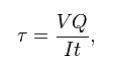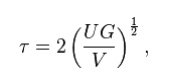# Beam shear

Beam shear is defined as the internal shear stress of a beam caused by the sheer force applied to the beam.

Beam shear

Beam shear is defined as the internal shear stress of a beam caused by the sheer force applied to the beam.where

V = total shear force at the location in question;

Q =  statical moment of area;

t = thickness in the material perpendicular to the shear;

I =  Moment of Inertia of the entire cross sectional area.

This formula is also known as the Jourawski formula

Semi-monocoque shear

Shear stresses within a  semi-monocoque structure may be calculated by idealizing the cross-section of the structure into a set of stringers (carrying only axial loads) and webs (carrying only  shear flows). Dividing the shear flow by the thickness of a given portion of the semi-monocoque structure yields the shear stress. Thus, the maximum shear stress will occur either in the web of maximum shear flow or minimum thickness.

Also constructions in soil can fail due to shear;  e.g., the weight of an earth-filled  dam or  dike may cause the subsoil to collapse, like a small  and slide.

Impact shear

The maximum shear stress created in a solid round bar subject to impact is given as the equation:where

U = change in kinetic energy;

G =  shear modulus;

V = volume of rod;Study Material, Lecturing Notes, Assignment, Reference, Wiki description explanation, brief detail
Mechanical : Strength of Materials : Torsion : Beam shear |